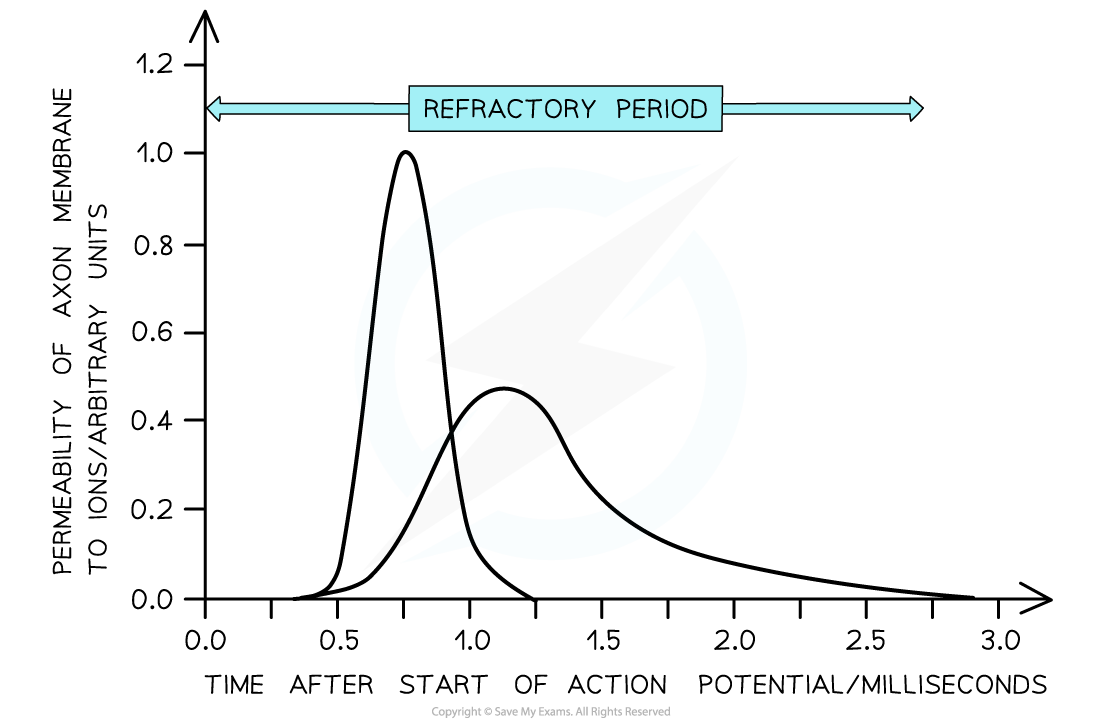# AQA A Level Biology复习笔记6.2.6 Maths Skill: Calculating Maximum Impulse Frequency

### Calculating the Maximum Frequency of Impulse Conduction

• After an action potential passes through a section of an axon it undergoes a period of recovery and is unresponsive
• This is known as the refractory period

• The refractory period means that there is a minimum time between action potentials occurring at any one place along a neurone
• The duration of the refractory period is key in determining the maximum frequency at which impulses can be transmitted along neurones (between 500 and 1000 per second)
• In order to find the maximum frequency of impulses within a certain period of time the following equation can be used:

Time ÷ duration of the refractory period

• Questions often ask for the maximum frequency of impulses within one second. This would make the equation:

1 ÷ duration of the refractory period

• Different units are used for these calculations
• Impulses sec-1
• Action potentials sec-1
• Hz (1 Hz is equal to one impulse per second)

#### Worked Example

Figure 1 shows the changes in the permeability of a section of an axon membrane to two ions that are involved in the production of an action potential. Use the information in Figure 1 to calculate the maximum frequency of action potentials per second along the axon. Show your working.Step 1: Determine the duration of the refractory period

From the graph: 2.75 milliseconds

Step 2: Convert this to seconds

0.00275 seconds

Step 3: Insert relevant figures into the equation

Time ÷ duration of the refractory period

1 ÷ 0.00275 = 363.63

Answer = 364 action potentials sec-1

#### Exam Tip

It is very important to check the units given in the question. For example, the duration of the refractory period may be given in milliseconds but the answer must be in action potentials sec-1. 1000 milliseconds = 1 seconds!